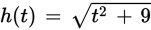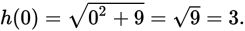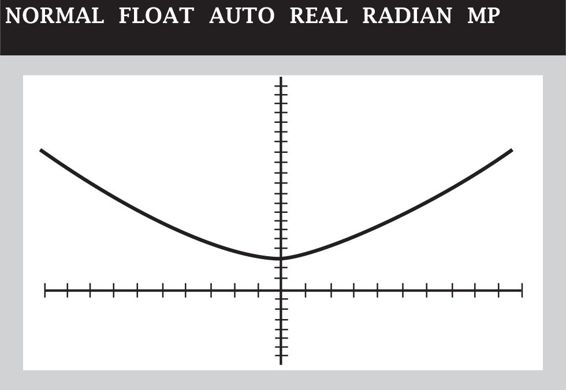# SAT Math Multiple Choice Question 376: Answer and Explanation

### Test Information

Question: 376

1. Iffor all real values of t, which of the following is not in the range of h(t)?

• A. 1
• B. 3
• C. 9
• D. 10

Explanation:

A

Difficulty: Medium

Category: Passport to Advanced Math / Functions

Strategic Advice: The range of a function is the set of possible outputs, or y-values on a graph.

Getting to the Answer: For all real values of any number t, the value of t 2 cannot be negative. This means the smallest possible value of t 2 is 0 and, consequently, the smallest possible value of h(t) isThus, the number 1 is not in the range of the function.

You could also graph the function in your graphing calculator and examine the possible y-values. The graph follows here:Notice that the lowest point on the graph is (0, 3), which tells you that the range of the function is h(t) ≥ 3.